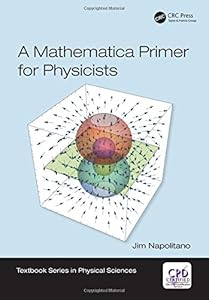# A Mathematica Primer for Physicists9781138035096

A Mathematica Primer for Physicists (Textbook Series in Physical Sciences)
by: Jim Napolitano
ISBN-10 书号： 1138035092
ISBN-13 书号： 9781138035096
Edition 版次： 1
Release Finelybook 出版日期： 2018-03-30
pages 页数： 214
Learn how to use Mathematica quickly for basic problems in physics. The author introduces all the key techniques and then shows how they’re applied using common examples. Chapters cover elementary mathematics concepts,differential and integral calculus,differential equations,vectors and matrices,data analysis,random number generation,animation,and visualization.
Written in an appealing,conversational style
Presents important concepts within the framework of Mathematics
Gives examples from frequently encountered physics problems
Explains problem-solving in a step-by-step fashion
Contents
Chapter 1 lntroduction
Chapter 2 Solving Algebraic Equations
Chapter 3 Derivatives,Integrals,and Series
Chapter 4 Differential Equations: Analytic Solutions
Chapter 5 Differential Equations: Numerical Solutions
Chapter 6 Vectors and Matrices
Chapter 7 Basic Data Analysis
Chapter 8 Fitting Data to Models
Chapter 9 Numerical Manipulations
Chapter 10 Random Numbers
Chapter 11 Animation
Chapter 12 Advanced Plotting and Visualization
Appendix B Shorthands

## 下载地址：

A Mathematica Primer for Physicists 9781138035096.pdf

### 评论 抢沙发

#### 支付宝扫一扫打赏#### 微信扫一扫打赏